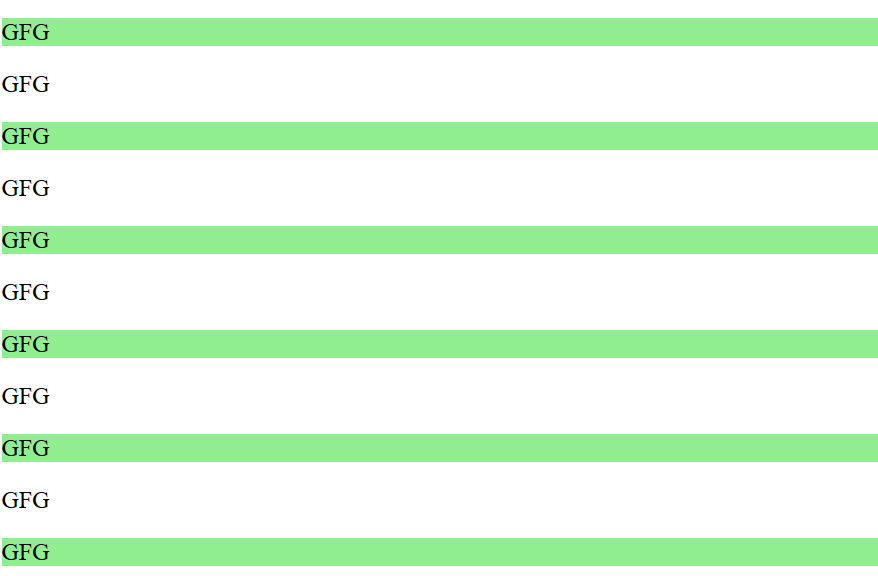Open In App

# jQuery :nth-child() Selector

The jQuery :nth-child() Selector Selects all elements that are the nth-child of their parent elements.

Syntax:

`\$("Selector:nth-child( index | even |odd |equation )")`
• Index: Index provided. Index starts from
• even: even number of child elements get selected.
• odd: odd number of child elements will get selected.
• equation: select child elements by solving a formula(an+b).

Example: In this example, we are using the above-explained nth-child() selector.

## HTML

 ```<``html``>` `<``head``>``    ``<``title``>jQuery :nth-child() Selector``    ``<``script` `src``=``"https://ajax.googleapis.com/ajax/libs/jquery/3.3.1/jquery.min.js"``>``    ````    ``<``script``>``        ``\$(document).ready(function () {``            ``\$("p:nth-child(2n-1)").css("background-color",``                ``"lightgreen");``        ``});``    ````` `<``body``>``    ``<``p``>GFG``    ``<``p``>GFG``    ``<``p``>GFG``    ``<``p``>GFG``    ``<``p``>GFG``    ``<``p``>GFG``    ``<``p``>GFG``    ``<``p``>GFG``    ``<``p``>GFG``    ``<``p``>GFG``    ``<``p``>GFG``` ``

Output: